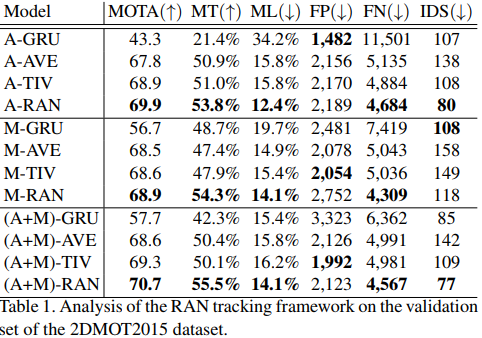The authors propose to formulate the multi-object tracking problem as a time series. To achieve that, they model each track by an autoregressive model (AR). In an AR model, each timestep is a weighted sum of the previous values plus some noise.

$x_{t} = \sum_{k = 1}^{K}w_{k}x_{t-k} + \epsilon_{t}$

To handle each track, the authors designed a Recurrent autoregressive network (RAN). (Fig. 2)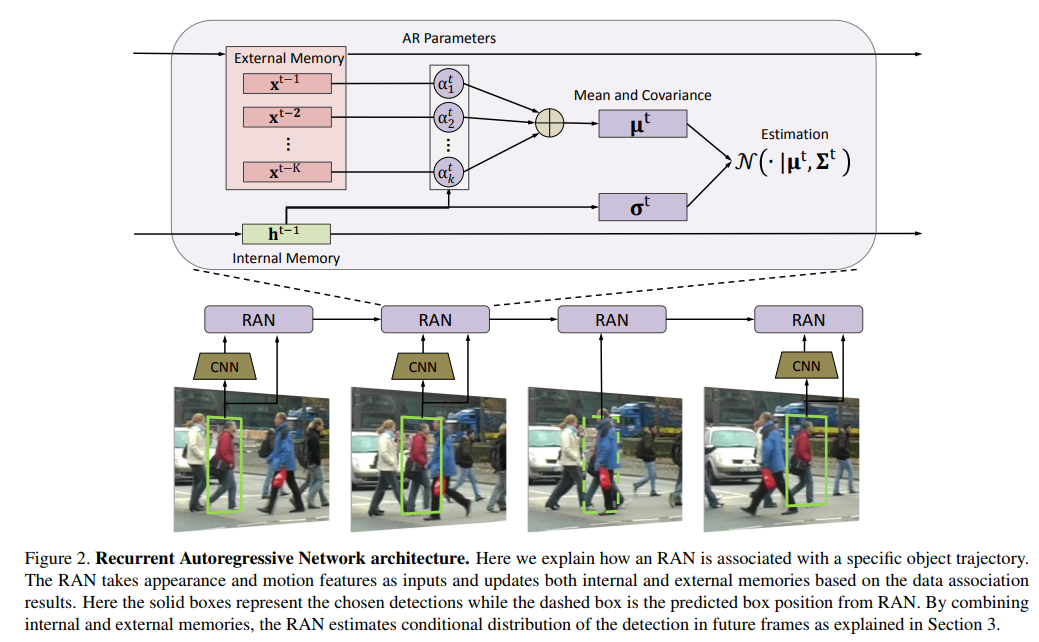This module estimates the probability that this detection is related to the current track. From appearance, motion features and the previous detections (external memory), RAN will predict wheter a new detection is associated with the current track $$P(x^{t}\vert x^{1:t-1})$$.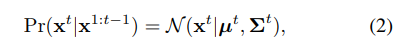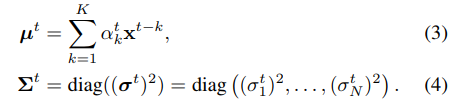For each frame, they assign each detection with a track or create new tracks for unassigned detections.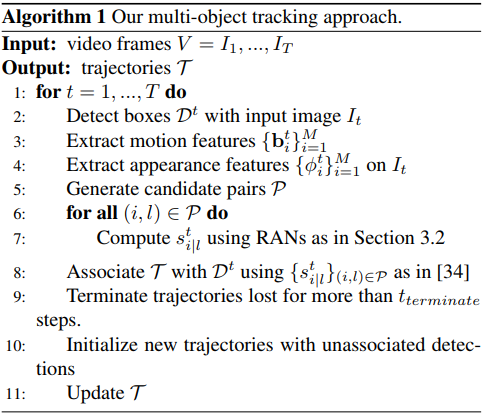The authors show great results on MOT2015 dataset. A draw back of this approach is that the tracks are often fragmented. They use Faster R-CNN to get their detections.